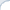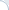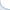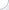Recently, I was discussing the problem of efficient exponentiation to integer powers. As I learned, the GNU library (apparently aiming for maximum precision) cannot carry out this operation efficiently. One can do better by doing exponentiation by squaring. What if the power is not integer, but fractional? The GNU library is working super slowly in this case and I cannot get more than miserly 20 million of exponentiations per second even on a modern Core i7.

What if I do not care about exponentiating efficiently for all the powers, but only for some rational ones? Can I do better? For example, I want to play with similarity search in pesky non-metric Lp spaces (for p < 1) and I can select only convenient values of p such as 1/2, 1/4, or 1/8. If I multiply these values by 8, I obtain integer numbers. One can see, and it is sure a very old idea, that we can use exponentiation by square rooting here. For instance, to compute the power 1/8, we can apply the square root three times. For the power 1/2 + 1/4, we need to obtain the square root and memorize it. Then, we apply the square root two more times and multiply the result by the already computed square root.

This algorithm relies on the binary representation of the exponent and works for the same reason as exponentiation by squaring. But this should be a crazy idea, because computing a square root is a costly operation, right? No, it is probably not. In many cases, square rooting takes about 2-3 CPU cycles and can be two orders of magnitude faster than the function pow!

I wrote a simple test to verify my hypothesis. As usual, I compile using both GCC and Intel. To prevent Intel and GNU from "cheating", I sum up all computed distances and print the result in the end. This is a good-enough trick for the GNU compiler, but not for the Intel compiler. If the variable sum becomes very large, the Intel-generated code is smart enough to stop computing powers, which defeats the purpose of testing. This is why I additionally adjust the variable sum through multiplying it by small constants. Adjustment operations do introduce little overhead, but it is very small compared to the cost of exponentiation. And, of course, we are careful enough not to use division:

    for (int j = 0; j < rep; ++j) {        for (int i = 0; i < N*4; i+=4) {            sum += 0.01 * pow(data1[i],   data2[i]);            sum += 0.01 * pow(data1[i+1], data2[i+1]);            sum += 0.01 * pow(data1[i+2], data2[i+2]);            sum += 0.01 * pow(data1[i+3], data2[i+3]);        }        sum *= fract;    }

Some benchmarking highlights:

1. In my test, exponentiation by squaring either matches performance of the GNU pow or is substantially faster;
2. When I plug the improved pow into the actual code for nearest neighbor search in pesky Lp (p<1) spaces, it provides more than a five-fold speed-up.
3. Exponentiation by squaring can even be faster than the Intel's function pow for exponent with less than 5 binary digits (if you compile the code using the Intel's compiler).

Notes on precision. This version produces results, which are almost identical to the results from the GNU function. If we can tolerate an approximate version (with a relative error about 10-5, there are more efficient solutions).